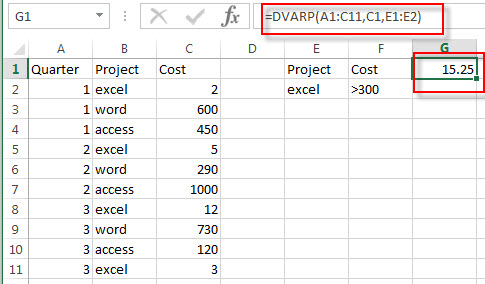## Excel DVARP Function

This post will guide you how to use Excel DVARP function with syntax and examples in Microsoft excel.

### Description

The Excel DVARP Function will get the variance of a population based on the entire population of numbers in a column in a list or database based on a given criteria. And the DVARP function can be used to evaluate text values and logical values in references in Excel.

The DVARP function is a build-in function in Microsoft Excel and it is categorized as a Database Function.
The DVARP function is available in Excel 2016, Excel 2013, Excel 2010, Excel 2007, Excel 2003, Excel XP, Excel 2000, Excel 2011 for Mac.

### Syntax

The syntax of the DVARP function is as below:

`= DVARP(database, field, criteria)`

Where the DVARP function arguments are:

• Database -This is a required argument. The range of cells that containing the database.
• Field – This is a required argument. The column with database that you want the minimum of.
• Criteria – The range of cells that contains the conditions that you specify.

### Excel DVARP Function Examples

The below examples will show you how to use Excel DVARP Function to get the variance of a population based on a sample by using the numbers in a column in a list or database that match a given criteria.

#1 to get the variance in the cost of excel project in a range A1:C11, using the following formula:

`=DVARP (A1:C11,C1,E1:E2)`### Related Functions

• Excel DVAR Function
The Excel DVAR Function will get the variance of a population based on a sample of numbers in a column in a list or database based on a given criteria.The syntax of the DVAR function is as below:= DVAR (database, field, criteria)…